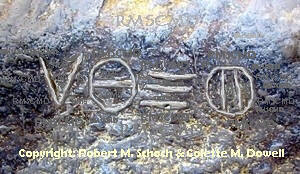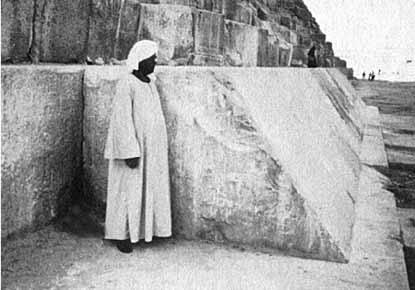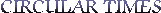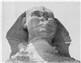The Inscription on the Entrance to the Great Pyramid of Khufu/Cheops

located in Giza, Egypt

This article is published for those of you who like to delve into mathematically encoded subjects that symbolically represent a supposed profound principle. This paper was conceived by a Mr. By J.Iuliano. This is his personal interpretation of the inscription that is on the main entrance to the Great Pyramid. No one seems to know what the inscription means and it has never been deciphered. I have received other notations on the inscription, but, hey, I am not that far out. Iuliano’s interpretation is as far out as I will contemplate, but, I think it has merit in the esoteric world. I thank all other entrees for this topic, I will still consider more ideas. These papers are of the opinion of the author only and are published for your enjoyment and inquiring mind.  Thank you, Colette

PS.....I  hope the diagrams lay out properly for the different browsers.My Interpretation of the Inscription on the Great Pyramid

Concerning the symbol at the gate of the great pyramid . vee(pyramid) , circle with a diameter line (c/d)= a circle with two vertical lines through it (Pi), represents the 2/Pi ratio of the great pyramid. ...article follows

What is the meaning of the Cheops pyramid? In reductionist terms , it has a shape (angle-form) , a measure (baseleg / height) in three dimensional space. These are its geometrical parameters and can describe its boundaries in form and measure , apex , base height , etc. As previously demonstrated these parameters can derive the electronic form , fine-structure...aem

aem =   ( .37 ^ 2 ) / ( 10 ^ ( 3.99999552 / Pi ))   = 1/137.03599911

....37 plays the active role here , as does the Cheops constructs...2*ht/bl = 4/Pi...But is this the simplest form for interpreting the object pyramid , that is,  height divided by base leg? Can it be simplified using a simpler contemplation ? If one could simplify the object to a single description , what would it be ? Observe this phenomenon of the Cheops face-angle...FA = 51.85 degrees Sumerian....(see references). A single expression that relates to exactly what occurs in the object , a slope projected into an apex. This is the single idea that Cheops represents , the idea of slope or angle and that this slope or angle representing this slope should contain everything in physical metrology as measured in the single fact of geometric angle... 51.85 degrees. Though the ht/bl ratio can demonstrate the fine-structure quite accurately using the Christian(37) form , the angular contemplation idea , however , or face-angle , can derive the single TOE equation...or unification of the four fundamental force constants of Nature  !!...aem-electromagnetic ; a(s) strong nuclear ; Gw-weak nuclear-fermi-couple ; Gn-gravitational constants and the minimum stable mass unit , the proton ..pmev. This single symbolic form , the face angle of the Cheops pyramid can describe to nine decimals of accuracy the metrology of modern physical measurement. This is demonstrated through two formulas derived from the universal equation for a black hole...Planck mass...Mp:

(( ev/Pi )^(4/3)) * (( h*c/E)^(2/3)) * 2.5 / (( Gf*as)^(1/3)) = Mp

( aem ^ 2 ) * Gn * ( 10 ^ 3 ) / a(s) / Gw = Mp

....the Cheops pyramid face-angle unites the two forms:

as / ( 37 ^ 3 ) / ( 10 ^ 9 )  / aem / Gn  /   Gw     /    pmev  =  51.85643356

aem / ( 37 ^ 3 ) / ( 10 ^ 6 )  /      Mp      / ( Gw ^ 2 ) / pmev  =  51.85643368

...interesting to note that Planck mass is a combination of gravity and electronics, that switching out the strong nuclear with electronic forms ,  forces the condensation of Planck mass and the squaring of the fermi-coupler (Gw) , energized , occurs:

short range
strong
a(s)
-----------------------------------------------------------------------------------   =  51.85643356
37^3 * 10^9   *   aem   *   Gn  *  Gw    *    pmev
electronic    gravity   weak        mass
long range   short range
\                 /
\      to      /
\ Planck  /
\  mass /
\        /
long range
electronic
aem
-------------------------------------------------------------------------------------   =  51.8564336
37^3 * 10^6        *      Mp     *   Gw^2  *  pmev
mass         weak        mass
short range

....note that the electronic form aem , is the switch to a field , going from denominator to numerator and that this action forces the black hole Planck mass to condense out of the interaction of the gravitational and electronic constants. The strong force , a(s) , is the coupler to the electronic gravity interaction. The unchanging constants are divine , being 10^6or9 , ( 37^3 ...a 3-d Christian King ) and the proton as minimum mass. Since these quantities remain unchanged in both equations , the main action is among the four fundamental forces. Inverting the as/aem ratio , the strong force and gravity convolute through their dimensional parameters, disappear and then reappear as the black hole entity,  Planck mass...Mp. One quantum unit of weak force Gw is created as the balancer to make the equation invariant to  the Cheops face angle: Action illustrated as:

as
-------------------                        begin (stable)
aem Gn Gw
l
l
l
aem                              create a field
-----------------------    interaction (invert as/aem) to fermi-coupler
as Gn Gw
l
l
l
1                               aem
-------------                    ----------------------      absorption(kicks out fermi-coupler)
as Gn -->--->---Gw--->---->Gw
l                                    l
l                                    l
l                                    l
1                                aem
------------          *        ----------------------            end (stable)
Mp                              Gw^2

Where does the electron fit into this scheme? If the proton is divine , is the electron also divine? The answer is incredibly yes!! The electron lies in the unchanging (divine) constants:

1 / ( 37 ^ 3 ) / pmev / ( 10 ^ 6 ) = 2.104098458 * ( 10 ^ - 14 )

...to extract the electron energy out of the equation above, one has to invert the 3-d Christian King(37) and wed to six powers of Egyptian Queen Isis(18):

( 666 ^ 6 ) / ( 37 ^ 3 ) / pmev / ( 10 ^ 6 ) = pmev / emev

...which reduce to:
( 37 ^ 3 ) * ( 18 ^ 6 ) / pmev / ( 10 ^ 6 ) =  pmev / emev
( 37 ^ 3 ) * (( 18 / 10 ) ^ 6 )                 = ( pmev ^ 2 ) / emev
( 37 ^ 3 ) * (( 18 / 10 ) ^ 6 ) / ( pmev ^ 2 ) = 1/emev
( pmev ^ 2 ) / ( 37 ^ 3 ) / (( 18 / 10 ) ^ 6 ) = emev = .510998918

...the equation predicts a value for the proton as:
pmev = 938.2749476 or 99.9997% of 2004 NIST values.

...the face-angle to electron relationship can be shown as the ratio....emev / FA:

( 10 ^ 15 ) * ( pmev ^ 3 ) * aem * GnGwa(s) / ( 18 ^ 6 ) = emev / FA

....this is the unification equation ; all four fundamental forces WITH the electron and proton energies linked in a single equation.

J.Iuliano

Mp = Planck mass = 2.176449594 * ( 10 ^ - 8 )kg ....exact
ev = electron volt = 1.602176527 * ( 10 ^ -19 )C.....exact
h = Planck's constant = 6.626069287 * ( 10 ^ - 34 ) J....exact
c = speed of light = 299792458 m/s....exact
E = permitivity of space = 8.854187818 * ( 10 ^ - 12 ) Fm....exact
Gf = 3.685789144 * ( 10 ^ - 82 ) Fm....exact
a(s) = strong force = 14.000436  ....~ 14
aem = fine-structure constant = 1 / 137.03599911....exact
Gw = fermi-coupler , weak force = .0000116638....exact
Gn = gravitational force =  6.67420559 * ( 10 ^ -11 ) m^3/kg/s^2...exact

THE SIGNIFICANCE OF THE GREAT PYRAMID'S SIDE ANGLE

Various degree angles have been postulated as the exact angle for the diagonal faces of the Great Pyramid and it is now generally accepted that the intended angle was very close to 51 degrees & 51 minutes. In a decimal rendition, this would equate to 51.85 degrees. The means whereby the angle has been estimated in modern times is based upon the few remaining weather worn casing stones at the base of the pyramid. Thankfully, one solitary course was buried under a high bank of sand during the era when the outer casing stones were being ravaged and plundered to build the city of Cairo

.Figure 4: The few solitary casing stones at the bottom of the Great Pyramid. These too would have been removed were it not for the good fortune that they were covered in tons of sand and inaccessible to those who plundered the Pyramid's stone to build the city of Cairo. These beautiful white limestone casing stones once sheathed the entirety of the Great Pyramid on every face, all the way up to its 44' X 44' flat floor altar on the top of the pyramid.

There are various degree angles given, all in the vicinity of 51.85 degrees, which different scientists have determined represent the intended, finite angle of the Great Pyramid.
Because of the weather worn and sand lashed condition of these limestone sheathed surfaces, the exact degree angle will never be resolved, definitively, by averaging the damaged angles of the casing stones themselves. We do, however, know that the architects were aiming for a figure around 51.85 degrees.

....  In the black hole singularity, the frequency of the surface area of the singularity is transferred to reality through frequency modes called, QNM's , quasi-normal modes, each with its own characteristic frequency and damping. In 1993 , Nollert  used computer calculations  to show that in the limit of large damping , the frequency (w) of these modes approaches a specific number depending only on the mass of the black hole:

w = .0437123 / mass of black hole

...plugging this result into a previous formula , Hod in 1998 , obtained the quantum of the area of any black hole mass : IN = reciprocal natural log e

quantum area = 4.394444...~ 4 * IN3

...later it was shown that the quantum area is indeed ...4*IN3...Symbolically the quantum area can be represented by one number as entity , this being 666 as energy of the black hole , and yet describe through mathematics, what the Nollert/Hod frequency constant ( .04371235) does exactly in the transition from black hole frequencies to electronic probabilities ( fine-structure constant ) and the laws of natural forms  , the golden mean -phi , pi , Feigenbaum etc., that control the electronic interactions that exist in the biological and static world forms. The incredible ability of the black hole frequency to interact as an exponential to the black hole energy...666, can be demonstrated by this amazing fact of invariance among ALL of the human measuring systems of the human mind.... trigonometry angle , measuring systems: the radian (2/Pi) , degree(360) and gradian(100) , this number being unique to performing this function. Starting with the Hod/Nollert black hole frequency constant as a function of black hole quantum area:

IN3 / 8 / Pi = .043712394 = Hod , Nollert discovery...1998

black hole frequency = Bhf = .0437123133..

IN( 80 * ( 666 ^ ( Bhf ^ 2 ))) = 4 * IN2.999999944 = quantum area BH

...the three human mathematical angle measuring systems and phi = 1.6180339875...

RADIANS.....cos(( 80 * ( 666 ^ ( Bhf ^ 2 ))) - 10 )  =  .61803394 / -2...
DEGREES...cos(( 80 * ( 666 ^ ( Bhf ^ 2 )))  - 9 )   =  .618034189 / 2...
GRADIANS..cos(( 80 * ( 666 ^ ( Bhf ^ 2 )))   - 1 )  =  .618034139 / 2...

...the golden mean , phi = 1.6180339875 , ( biological form rules ) remains invariant among all systems of measurement. I can't find another number that does this !!!  Another mathematical trick of the black hole entity is the fine-structure constant derived from black hole energy only...this is the collective unconscious form:  (( 80 * ( 666 ^ ( Bhf ^ 2 ))) - 9 ) * 36 = Plato = 2592

666 / ( 10 ^ (((( 80 * ( 666 ^ ( Bhf ^ 2 ))) - 9 ) * 36 ) / 666 )) = sqrt aem

(  666 ^ 2 ) / (10 ^ ( 287.999975837 / 37 ))  =  aem = 1/137.035999701 = fine-structure constant

...note the use of the degree form (72) for translation to fine-structure ...this is ancient, ancient , ancient knowledge !!

80 * ( 666 ^ ( Bhf ^ 2 )) - 9   =    71.99999396  ~   72

....288 is the Kabballic  genesis number...all matter originating from ---

" 288 sparks from broken vessels "....

By J.Iuliano

Cabbala Kabala cabala cabbalaThe Official Website of

Dr. Colette M. Dowell & Dr. & Robert M. Schoch, Ph.D.An International Networking Educational Institute

Intellectual, Scientific and Philosophical Studies

Angela Praxter - Professional AssistantThe Official Website of

Dr. Robert M. Schoch, Ph.D.